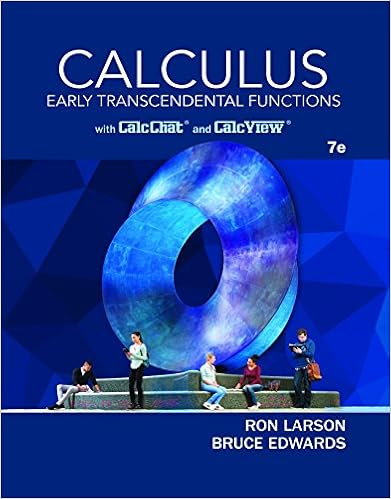# W6_Lab_Worksheet - MATH260Week 6 Lab Name Nirfana Elshahawi...

• Lab Report
• 12
• 100% (7) 7 out of 7 people found this document helpful

This preview shows page 1 - 3 out of 12 pages.

##### We have textbook solutions for you!
The document you are viewing contains questions related to this textbook.The document you are viewing contains questions related to this textbook.
Chapter 7 / Exercise 14
Calculus: Early Transcendental Functions
Edwards/LarsonExpert Verified
MATH260—Week 6 LabName: Nirfana Elshahawi Antiderivatives According to the first part of the fundamental theorem of calculus, the antiderivative reverses the derivative.If f(x) is a derivative, F(x) is the antiderivative.Directions:Look at the examples below and answer questions 1 and 2.Let f(x) be a derivative and F(x) be the anitderivative.a.)f(x) = 3x2b.)f(x) = 5x – 63
x
4
42) Find the antiderivative of f(x) = 3x5+ x – 4. Show all work.
##### We have textbook solutions for you!
The document you are viewing contains questions related to this textbook.The document you are viewing contains questions related to this textbook.
Chapter 7 / Exercise 14
Calculus: Early Transcendental Functions
Edwards/LarsonExpert Verified
4) What do the derivatives of the following antiderivativeshave in common?
– 11
Directions:Look at the examples, then find each of the integrals below.
C
x
•••